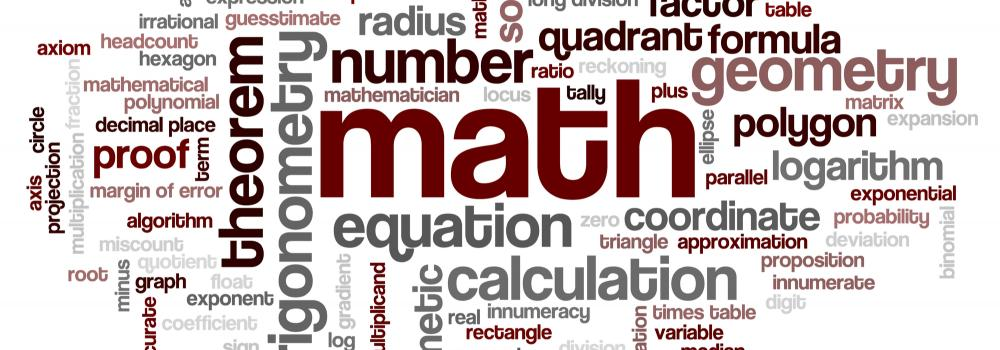# Secondary/7-9: Other Maths TopicsHere you will find other mathematical concepts that do not fit easily with the topic but are important in developing a rounded mathematician.

### Bronze

• The Order of Calculations
• Prime Numbers
• Cubes and Cube Roots
• Factors and Multiples
• Prime Factors
• Highest Common Factor
• Lowest Common Multiple
• Rounding Decimals
• Any Percentage of any Number
• Addition and Subtraction of Decimals

### Silver

• Special Numbers
• Lines and Angles
• Powers
• Positive Indices
• Negative Indices
• Calculating with Standard Form
• Discrete Data
• Continuous Data
• Pie Charts
• Averages
• Experimental probability
• Probability of two or More Events
• Subtracting Fractions

### Gold

• Finding the Midpoint of a Line
• Translation
• Reflection
• Rotation
• Enlargement
• Multi-transformations
• Straight Line Graphs